# Chapter 3 OWL Deadline 27 September All material

• Slides: 29Chapter 3 • OWL Deadline 27 -September – All material through Chapter 3 is fair game for Exam #1 on 24 -September. • Lab the week of 10 -September: Synthesis of Alum • Stoichiometry and Equations – – – The Mole Stoichiometry and Compounds Chemical Reactions Limiting Reactants % and Theoretical YieldWhat is a mole? • A standard unit of quantity. • The number of atoms present in exactly 12 grams of 12 C. • Avogadro’s number is the constant for 1 mole • NA = 6. 02 x 1023Stoichiometry & Compounds • In terms of compounds, stoichiometry refers to the ratio of elements within a molecule or substance (including polyatomic ions and ionic compounds) • Any unique compound will have its formula • The formula will have a specific ratio of atoms • These ratios can be expressed in weight (grams for example) or quantity (moles usually) • H 2 O • C 3 H 8 • PO 4 -3 • H 2 C 2 O 4 2 H 2 O • Na 2 CO 3• Formula Mass (weight) • The sum of the atomic masses of all atoms in a formula unit of the compound, whether molecular or not. – Empirical Formula Mass – Molecular Formula Mass (another term for molecular mass) – The mass of some other unit containing multiple atoms • Molar Mass (weight) – The sum of the masses of all of the atoms or molecules in a mole of a compound, ordinarily expressed in grams/mole. Copyright © Cengage Learning. All rights reserved. 3|4Ca(OH)2 1 Ca 1(40. 08) = 2 O 2(16. 00) = 2 H 2(1. 008) = Total 40. 08 amu 32. 00 amu 2. 016 amu 3 significant figures 74. 095 74. 1 amu Calculate the formula weight of the following compound from its formula. Report your answers to three significant figures. Calcium hydroxide, Ca(OH)2 Copyright © Cengage Learning. All rights reserved. 3|5• A sample of nitric acid, HNO 3, contains 0. 253 mol HNO 3. How many grams is this? First, find the molar mass of HNO 3: 1 H 1 N 3 O 1(1. 008) = 1(14. 01) = 3(16. 00) = Copyright © Cengage Learning. All rights reserved. 1. 008 14. 01 48. 00 63. 018 3|7 (2 decimal places) 63. 02 g/mol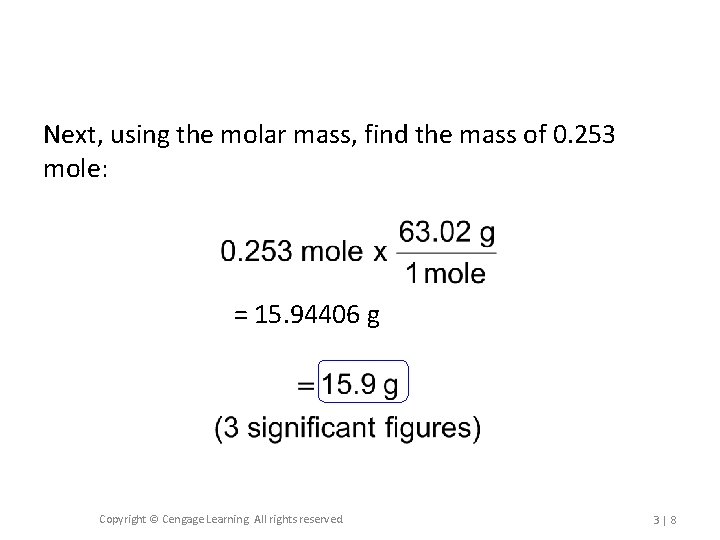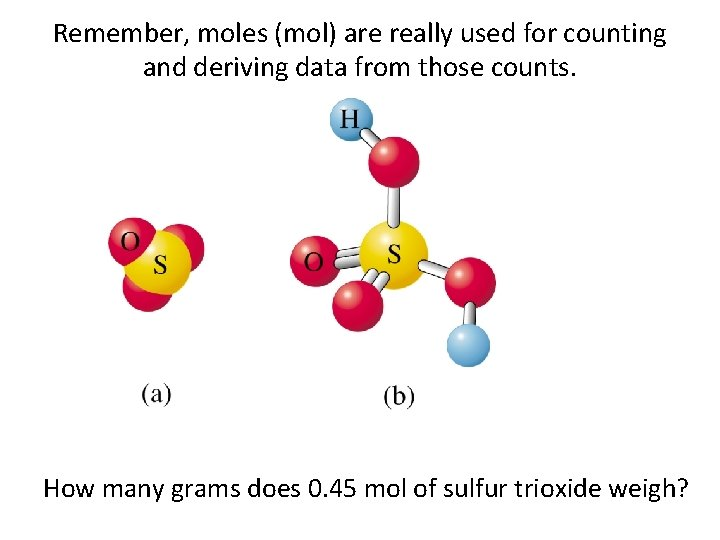Remember, moles (mol) are really used for counting and deriving data from those counts. How many grams does 0. 45 mol of sulfur trioxide weigh?Percent Composition • Often used in chemical analysis and identifying empirical or molecular formulas • A weight ratio of the elements in a compound • Write the name of, identify the stoichiometry involved in, and determine the % composition of each element in • Mg. CO 3Empirical/Molecular Formulas and % Composition • Chemists may discover a compound and, if it is new to them, have no idea what the chemical formulas are – Empirical – Molecular • You can use a step-by-step approach to determine the Empirical and Molecular formulas even if you have limited data. • Determine % composition (wt %) of each element. • Calculate the mole ratio for each element. – Round up to whole numbers • Use the molecular weight and the empirical formula weight to calculate the molecular formula – We estimate, pretty closely, the molecular weight from techniques such as mass spectrometry• You’ve discovered what you think is a new compound: – 2. 00 grams of it were found – Chemical analysis shows that it has Carbon and Hydrogen • What kind of compound is it? – Chemical analysis shows that of the sample that you have, 0. 151 grams of Hydrogen. – Mass spectrometry shows that the molecular weight is 26. 02 grams/mole – What compound is it?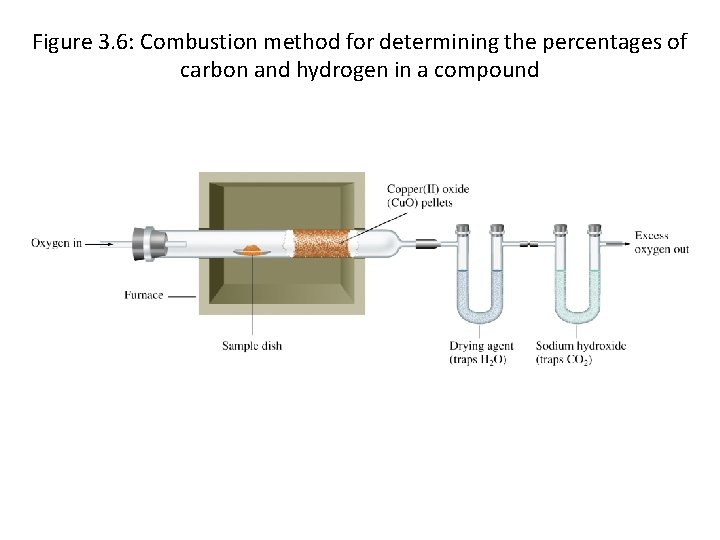Figure 3. 6: Combustion method for determining the percentages of carbon and hydrogen in a compound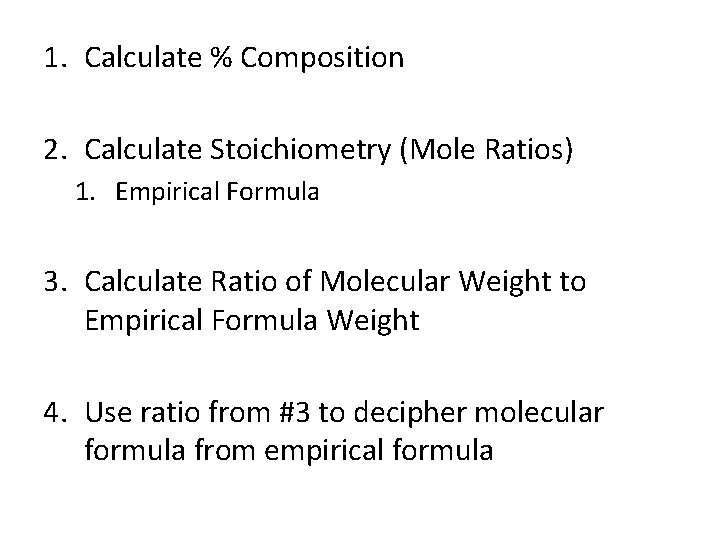1. Calculate % Composition 2. Calculate Stoichiometry (Mole Ratios) 1. Empirical Formula 3. Calculate Ratio of Molecular Weight to Empirical Formula Weight 4. Use ratio from #3 to decipher molecular formula from empirical formula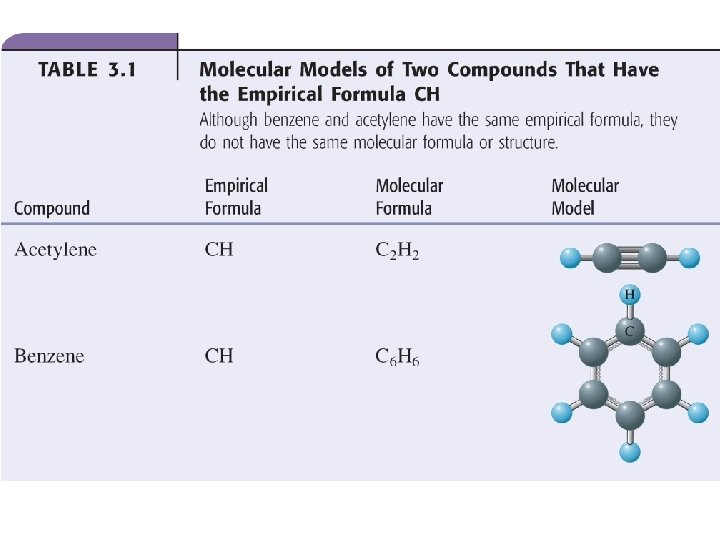Stoichiometry and Reactions • Chemical reactions take place with defined ratios of reactants and products • These defined ratios are based on the stoichiometry of the compounds or atoms in the reaction, and the stoichiometry related to the reaction itself. – Stoichiometric coefficients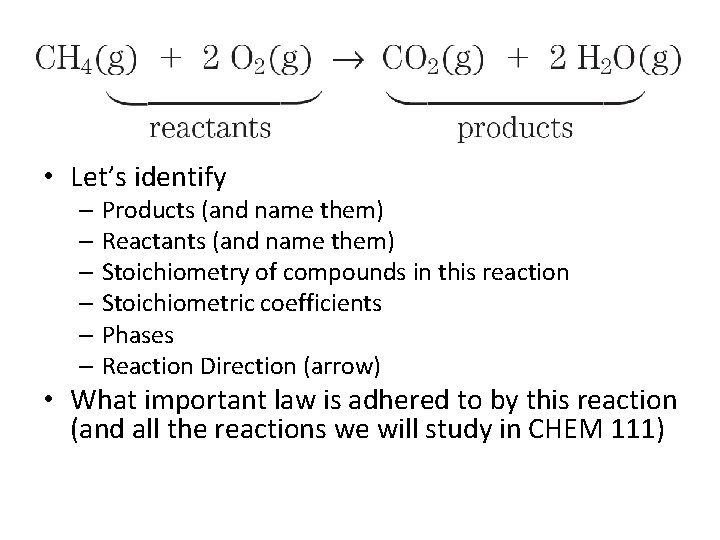• Let’s identify – Products (and name them) – Reactants (and name them) – Stoichiometry of compounds in this reaction – Stoichiometric coefficients – Phases – Reaction Direction (arrow) • What important law is adhered to by this reaction (and all the reactions we will study in CHEM 111)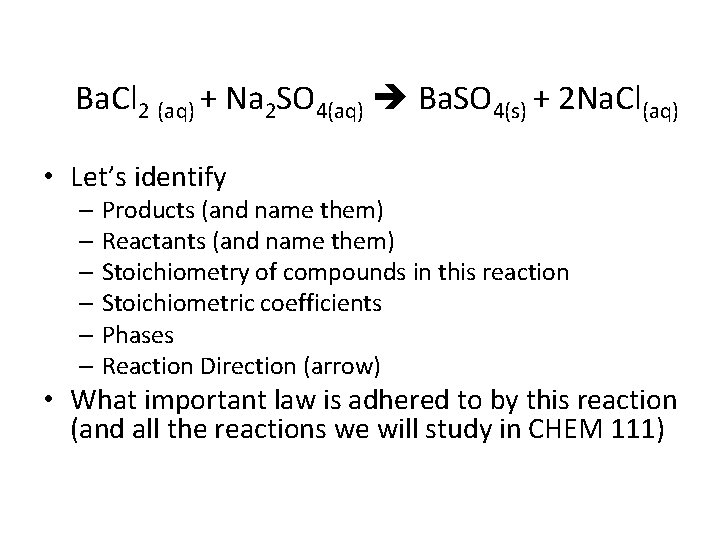Ba. Cl 2 (aq) + Na 2 SO 4(aq) Ba. SO 4(s) + 2 Na. Cl(aq) • Let’s identify – Products (and name them) – Reactants (and name them) – Stoichiometry of compounds in this reaction – Stoichiometric coefficients – Phases – Reaction Direction (arrow) • What important law is adhered to by this reaction (and all the reactions we will study in CHEM 111)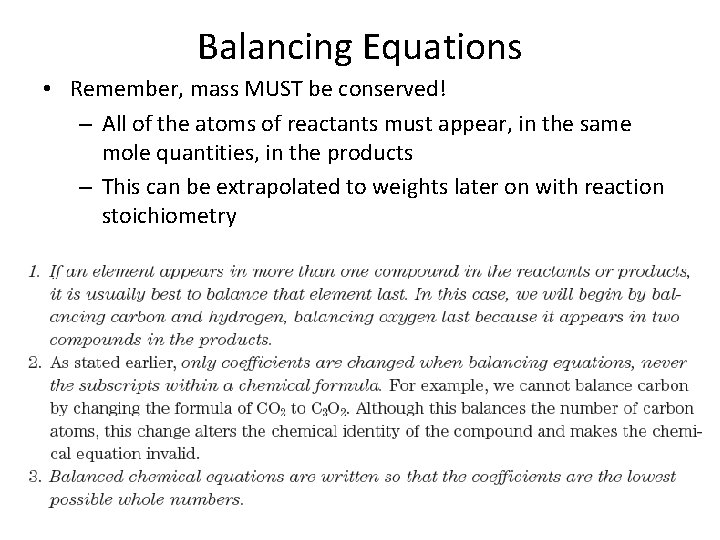Balancing Equations • Remember, mass MUST be conserved! – All of the atoms of reactants must appear, in the same mole quantities, in the products – This can be extrapolated to weights later on with reaction stoichiometry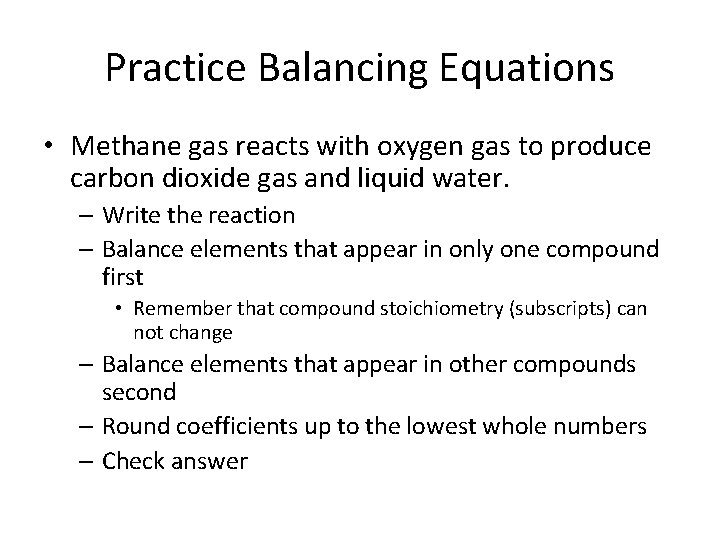Practice Balancing Equations • Methane gas reacts with oxygen gas to produce carbon dioxide gas and liquid water. – Write the reaction – Balance elements that appear in only one compound first • Remember that compound stoichiometry (subscripts) can not change – Balance elements that appear in other compounds second – Round coefficients up to the lowest whole numbers – Check answer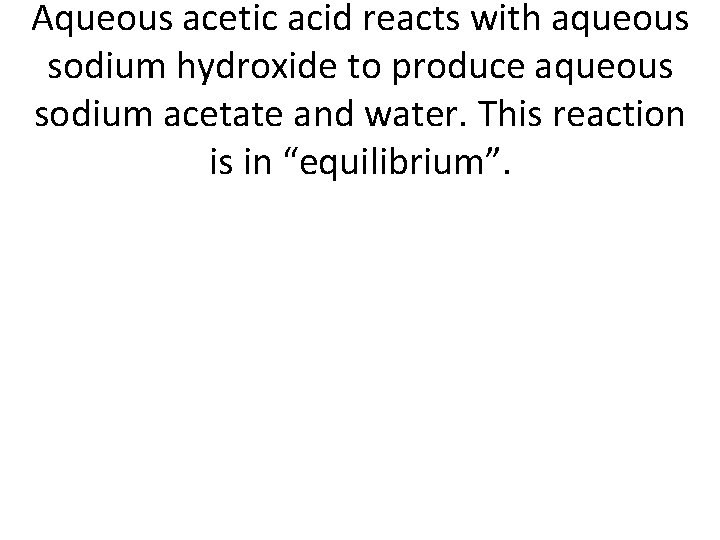Aqueous acetic acid reacts with aqueous sodium hydroxide to produce aqueous sodium acetate and water. This reaction is in “equilibrium”.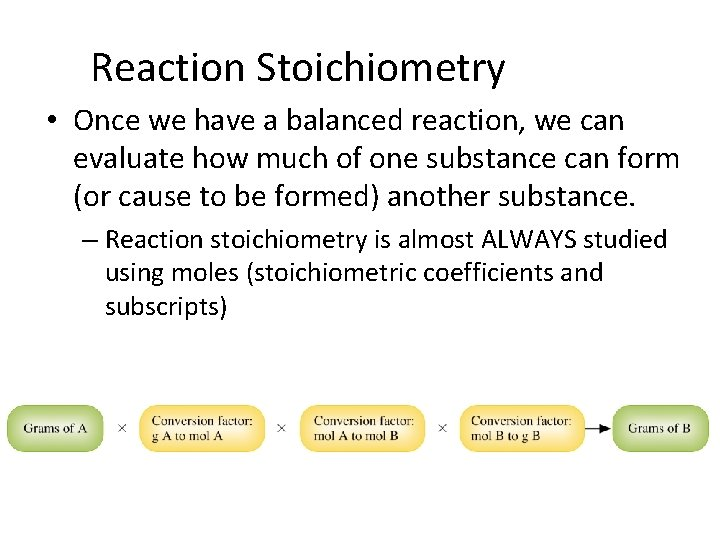Reaction Stoichiometry • Once we have a balanced reaction, we can evaluate how much of one substance can form (or cause to be formed) another substance. – Reaction stoichiometry is almost ALWAYS studied using moles (stoichiometric coefficients and subscripts)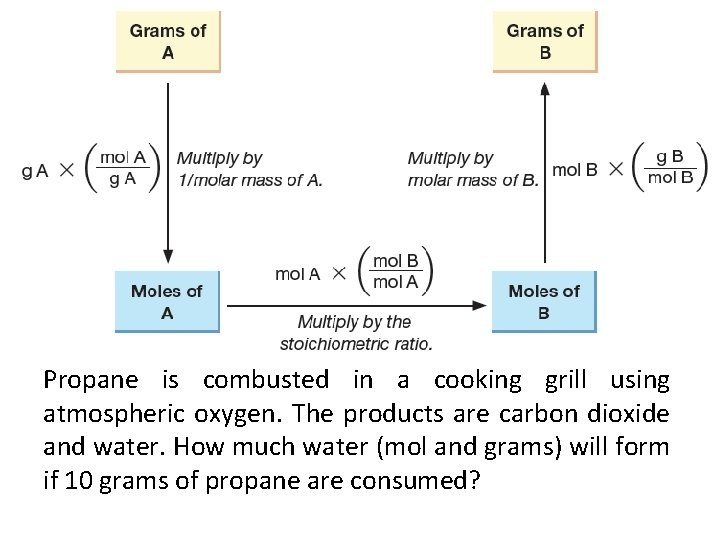Propane is combusted in a cooking grill using atmospheric oxygen. The products are carbon dioxide and water. How much water (mol and grams) will form if 10 grams of propane are consumed?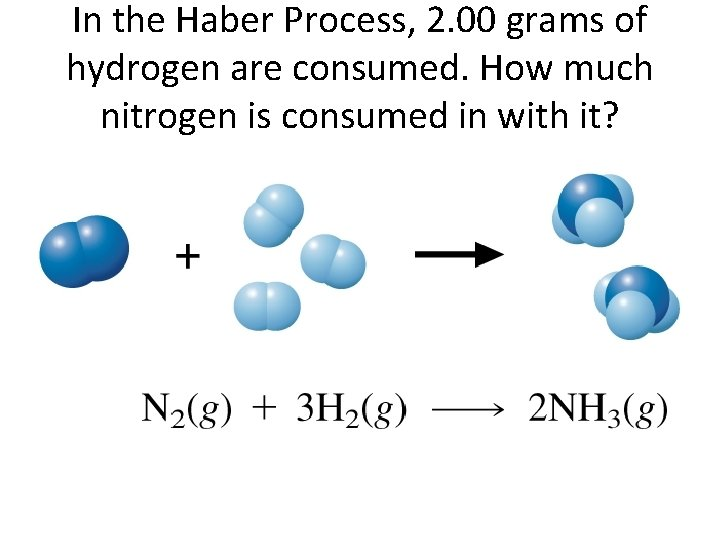In the Haber Process, 2. 00 grams of hydrogen are consumed. How much nitrogen is consumed in with it?Limiting Reactants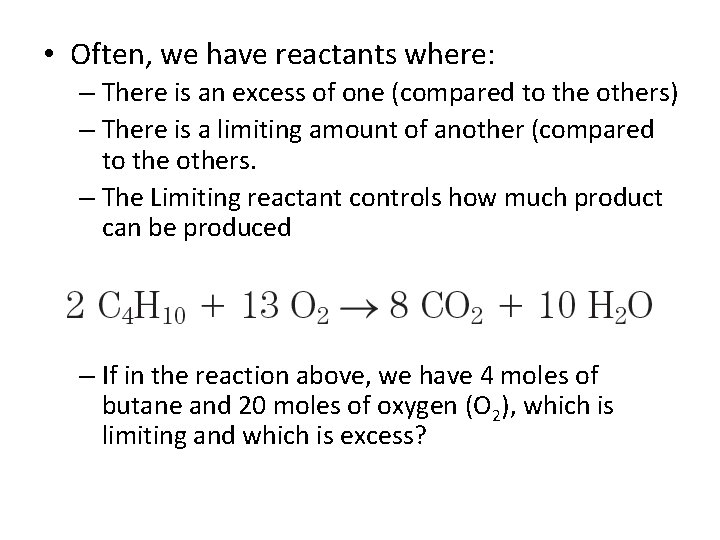• Often, we have reactants where: – There is an excess of one (compared to the others) – There is a limiting amount of another (compared to the others. – The Limiting reactant controls how much product can be produced – If in the reaction above, we have 4 moles of butane and 20 moles of oxygen (O 2), which is limiting and which is excess?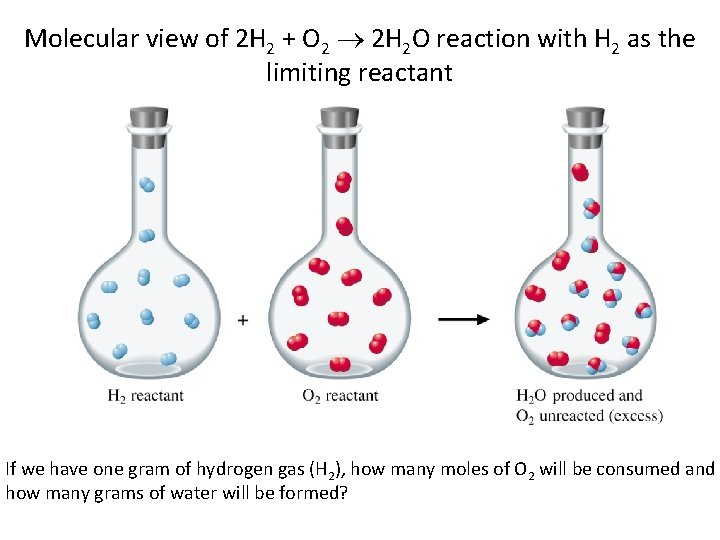Molecular view of 2 H 2 + O 2 2 H 2 O reaction with H 2 as the limiting reactant If we have one gram of hydrogen gas (H 2), how many moles of O 2 will be consumed and how many grams of water will be formed?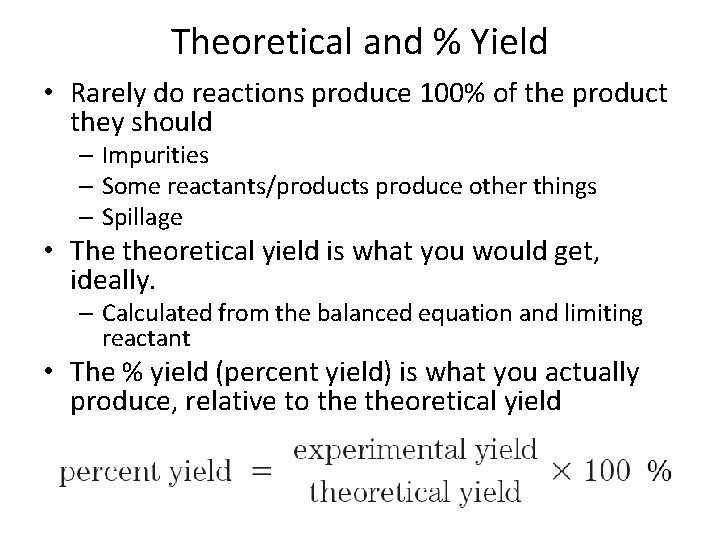Theoretical and % Yield • Rarely do reactions produce 100% of the product they should – Impurities – Some reactants/products produce other things – Spillage • The theoretical yield is what you would get, ideally. – Calculated from the balanced equation and limiting reactant • The % yield (percent yield) is what you actually produce, relative to theoretical yield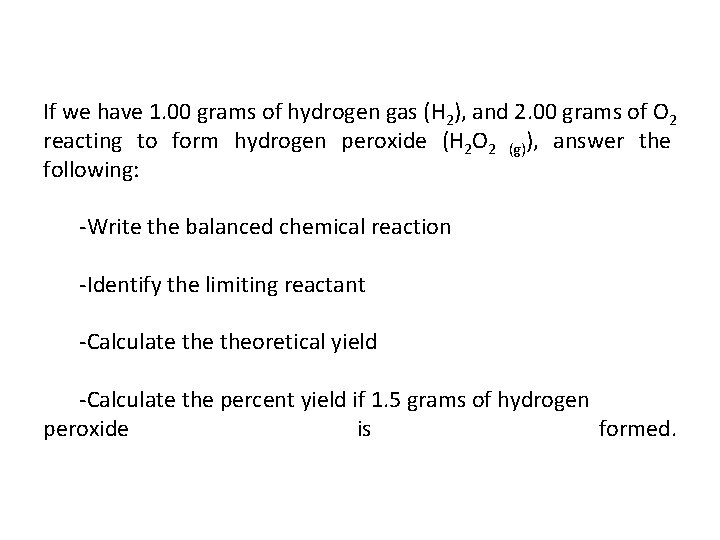If we have 1. 00 grams of hydrogen gas (H 2), and 2. 00 grams of O 2 reacting to form hydrogen peroxide (H 2 O 2 (g)), answer the following: -Write the balanced chemical reaction -Identify the limiting reactant -Calculate theoretical yield -Calculate the percent yield if 1. 5 grams of hydrogen peroxide is formed.